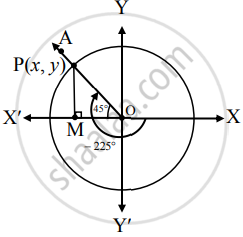# Find the trigonometric functions of : −225° - Mathematics and Statistics

Sum

Find the trigonometric functions of :

−225°

#### Solution

Angle of measure (– 225°):Let m∠XOA = −225°

Its terminal arm (ray OA) intersects the standard unit circle at P(x, y).

Draw seg PM perpendicular to the X-axis

∴ ΔOMP is a 45° – 45° – 90° triangle.

OP = 1,

OM = 1/sqrt(2)"OP"

= 1/sqrt(2)(1)

= 1/sqrt(2)

PM = 1/sqrt(2)"OP"

= 1/sqrt(2)(1)

= 1/sqrt(2)

Since point P lies in the 2nd quadrant,

x < 0, y > 0

∴ x = –  OM = (-1)/sqrt(2) and y = "PM" = 1/sqrt(2)

∴ P ≡ ((-1)/sqrt(2), 1/sqrt(2))

sin (– 225°) = y = 1/sqrt(2)

cos (– 225°) = x = -1/sqrt(2)

tan (– 225°) = y/x = (1/sqrt(2))/(-1/sqrt(2)) = – 1

cosec (– 225°) = 1/y = 1/((1/sqrt(2))) = sqrt(2)

sec (– 225°) = 1/x = 1/((-1/sqrt(2))) = -sqrt(2)

cot (– 225°) = x/y = (-1/sqrt(2))/(1/sqrt(2)) = – 1

Concept: Trigonometric Functions of Specific Angles
Is there an error in this question or solution?